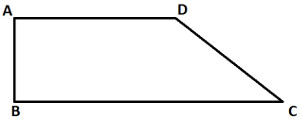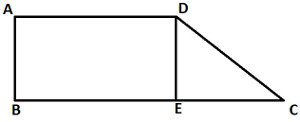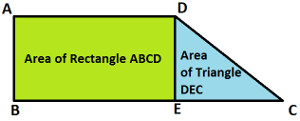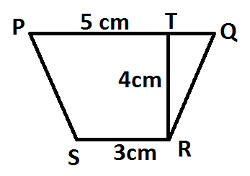Arithmetic
Associative Property
Averages
Brackets
Closure Property
Commutative Property
Conversion of Measurement Units
Cube Root
Decimal
Distributivity of Multiplication over Addition
Divisibility Principles
Equality
Exponents
Factors
Fractions
Fundamental Operations
H.C.F / G.C.D
Integers
L.C.M
Multiples
Multiplicative Identity
Multiplicative Inverse
Numbers
Percentages
Profit and Loss
Ratio and Proportion
Simple Interest
Square Root
Unitary Method
Algebra
Algebraic Equation
Algebraic Expression
Cartesian System
Linear Equations
Order Relation
Polynomials
Probability
Standard Identities & their applications
Transpose
Geometry
Basic Geometrical Terms
Circle
Curves
Angles
Define Line, Line Segment and Rays
Non-Collinear Points
Parallelogram
Rectangle
Rhombus
Square
Three dimensional object
Trapezium
Triangle
Trigonometry
Trigonometry Ratios
Data-Handling
Arithmetic Mean
Frequency Distribution Table
Graphs
Median
Mode
Range
Home >> Trapezium >> Area of Trapezium >>

## Area of Trapezium

 Area of Trapezium

 Before you study, how to find area of trapezium, you are advised to read: What are Parallel Lines ? How to find Area of Triangle ? How to find area of Rectangle ? Formula for calculating Area of Trapezium: Area of Trapezium = Height ( Σ of || lines ) / 2 Also, note that area of trapezium is always in unit2 e.g. cm2 or m2 etc….. Now, let's understand how formula for area of trapezium is obtained: Observe the following diagram:Above diagram represent Trapezium ABCD. Let's draw a line segment DE parallel to side AB (as shown below)You can observe that here we got a rectangle ABCD and a triangle DEC Now, recall formula for area of Rectangle and Area of Triangle & apply as shown below: Area of Rectangle ABCD = Length X Breadth Since length = AD and Breadth = DE, we get: Area of Rectangle ABCD = AD X DE ……………………..(statement 1) Area of Triangle DEC = 1/2 (Base X Height) Since Base = EC and height = DE, we get: Area of Triangle DEC = 1/2 (EC X DE) Now, Area of Trapezium is equal to area of rectangle ABCD and area of triangle DEC; as shown below:So we get: Area of Trapezium ABCD = Area of Rectangle ABCD + Area of Triangle DEC Put the values from above statement 1 & 2 and we get: = [ AD X DE ] + [ 1/2 ( EC / DE ) ] Take DE common and we get: = DE [ AD + 1/2 EC ] Balance the term in the bracket and we get: = DE [ 2AD + EC ] / 2 Or we can write it as: = DE [ AD + AD + EC ] / 2 Since AD = BE (opposite sides of rectangle are equal), so we get: = DE [ AD + BE + EC ] / 2 See the diagram again, BE + EC = BC, so we get: = DE [ AD + BC ] / 2 Now DE = Height , AD and BC are parallel lines, so we get required formula of trapezium: Height ( Σ of || lines ) / 2 Let's use the formula to following example: Example : Find the area of following Trapezium:Solution: In the given diagram: PQ and SR are parallel line of trapezium PQRS PQ = 5 cm SR = 3 cm TR is the height of trapezium PQRS TR = 4 cm Apply the formula: Area of Trapezium = Height ( Σ of || lines ) / 2 Or we can write its as: = TR (PQ + SR) / 2 Put values of TR, PQ and SR from the above and we get: = 4 ( 5 + 3 ) / 2 Solve brackets and we get: = 4 (8) / 2 Solve division expression and we get: = 4 (4) Solve multiplication expression and we get: = 16 Hence, area of trapezium PQRS is 16 cm2

Terms & Conditions